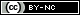## Artigo

•Citado por SciELO
•Acessos

•Similares em SciELO

## versão impressa ISSN 1665-2738

#### Resumo

HERNANDEZ-MARTINEZ, E.; VALDES-PARADA, F.J.  e  ALVAREZ-RAMIREZ, J.. Integral formulations for generalized reaction-diffusion equations. Rev. Mex. Ing. Quím [online]. 2011, vol.10, n.3, pp.363-371. ISSN 1665-2738.

Integral equation formulations are methodologies that consists on transforming the differential operators into integral operators exactly incorporating the boundary conditions. This work extends the use of integral equations formulations (IEF) for the development of numerical schemes for generalized reaction-diffusion systems. Due to the property of exact incorporation of the boundary conditions, the numerical schemes resulting do not require approximations at the boundaries, which leads to numerical schemes with global orders approximation O(h2). IEF schemes are characterized by their systematic methodology and its their mathematical structure that allows easy physical interpretation of the processes involved in the reaction-diffusion systems. To evaluate the ability of numerical approximation of the proposed scheme different conditions that arise in the study of catalytic pellets were considered. The numerical simulations carried out show that the IEF exhibit better numerical approximations than classic finite differences schemes (FD).

Palavras-chave : reaction-transport system; Green's function; numerical solution.

· resumo em Espanhol     · texto em Espanhol     · Espanhol (pdf )Todo o conteúdo deste periódico, exceto onde está identificado, está licenciado sob uma Licença Creative Commons